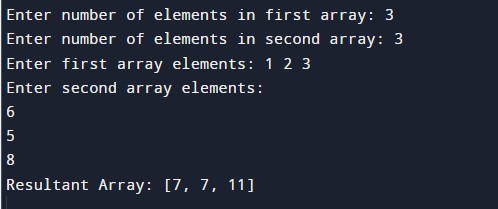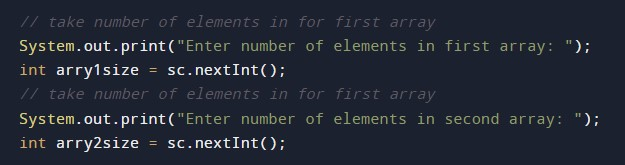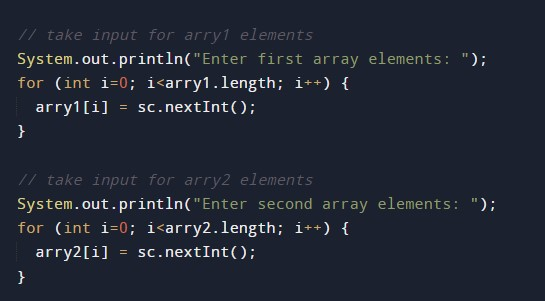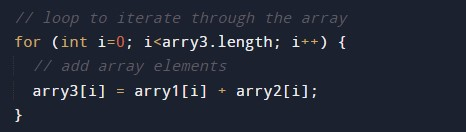# Java Program to find Sum of Two Arrays Elements

In this tutorial you will learn about the Java Program to find Sum of Two Arrays Elements and its application with practical example.

## Java Program to find Sum of Two Arrays Elements

In this tutorial, we will learn to create a Java program that will Find the Sum of Two Arrays Elements using Java programming.

## Prerequisites:-

Before starting with this tutorial, we assume that you are the best aware of the following Java programming topics:

• Operators in Java Programming.
• Basic Input and Output function in Java Programming.
• Basic Java programming.
• For loop in Java programming.
• Arithmetic operations in Java Programming.

## Program description to Find Sum of two array Elements.

In this program, we will first take the input array size of both arrays then we will check the size of both arrays. Now we will take the elements of both the array. Then we will add all the elements of both the array using the for a loop. At last, we will print that sum of both the arrays element using the print function.

With the help of this program, we can Find the Sum of Two Arrays Elements.

## Output:-In the above program, we have first initialized the required variable.• arry1[] = it will hold the integer value of the input elements.
• arry2[] = it will hold the integer value of the input elements.
• i = it will hold the integer value.
• arry3[] = it will hold the integer value sum of the array.

Input size of the array 1 and 2 from the user.Taking the elements of the array from the user.Program Code to find the sum of two array elements.Printing output sum of the two array elements.In this tutorial we have learn about the Java Program to find Sum of Two Arrays Elements and its application with practical example. I hope you will like this tutorial.